Cylindrical and spherical spreading are two simple approximations used to describe how sound level decreases as a sound wave propagates away from a source.

Spherical spreading describes the decrease in level when a sound wave propagates away from a source uniformly in all directions. This situation occurs for a sound source at mid-depth in the ocean, for example. One can picture the crests and troughs of the sound waves as spheres centered on the source location.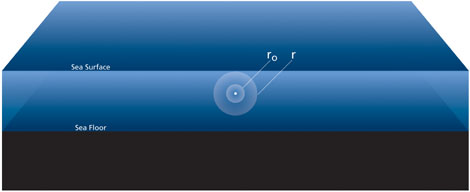Sound generated by a sound source (shown as a white dot) at mid-depth in the ocean is radiated equally in all directions. Sound levels are therefore constant on spherical surfaces surrounding the sound source. Sound levels decrease rapidly as sound spreads out from a sphere with a radius of r0 to a larger sphere with a radius r.

The rate at which intensity decreases for spherical spreading can be obtained using the definition of intensity and the principle of the conservation of energy. Intensity is the average amount of sound energy transmitted per unit time through a unit area in a specified direction. The amount of energy per unit time is power, and intensity is therefore the amount of power transmitted through a unit area in a specified direction. (For simplicity, the magnitude of the intensity is often referred to as the intensity, without specifying the direction in which the sound is traveling.) If the acoustic power generated by the source is radiated equally in all directions, it must be distributed equally over the surface of any sphere surrounding the source. The total power crossing any sphere surrounding the source therefore equals the intensity times the area of the sphere:$P=4 \pi r^2 I$

In this expression P is the total power, r is the radius of the sphere, and I is the intensity. If there is no sound absorption in the medium, then the total power crossing all such spheres is the same:$P=4 \pi r^2_{0} I_{0} = 4 \pi r^2 I$

Solving for I gives: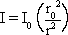Scientists say that the intensity decreases as the inverse square of the range for spherical spreading. If we pick r0 to be 1 meter, then I0 is just the acoustic source level. The amount by which the intensity decreases relative to its level at the source is called the transmission loss. Transmission loss is usually expressed in decibels: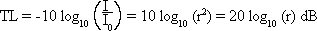We can now construct a table showing the relative intensity levels and transmission losses for spherical spreading at various ranges, assuming that r0 is 1 meter.

 Range, r (meters) Relative Intensity, I / I0 Transmission Loss, TL (dB) 1 1 0 10 1/100 20 100 1/10,000 40 1000 1/1,000,000 60

The relative intensity level decreases rapidly for spherical spreading. Equivalently, the transmission loss increases rapidly.

Sound cannot propagate uniformly in all directions from a source in the ocean forever. Beyond some range the sound will hit the sea surface or sea floor. A simple approximation for spreading loss in a medium with upper and lower boundaries can be obtained by assuming that the sound is distributed uniformly over the surface of a cylinder having a radius equal to the range r and a height H equal to the depth of the ocean.Sound generated by a source (shown as a white dot) in mid-ocean cannot continue to spread uniformly in all directions once it reaches the sea surface or sea floor. Once the sound is trapped between the top and bottom of the ocean it gradually begins to spread cylindrically, with sound radiating horizontally away from the source. Sound levels decrease more slowly as sound spreads from a cylinder with a radius of r0 to a larger cylinder with radius r compared with the rate of decrease for spherical spreading.

The total power crossing a cylinder surrounding the source equals the intensity times the area of the cylinder: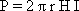If there is no sound absorption in the medium, then the total power crossing all such cylinders is the same: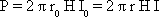Solving for I gives: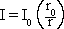Scientists say that the intensity decreases as the inverse first power of the range for cylindrical spreading. If we pick r0 to be 1 meter, then I0 is the source level. The transmission loss in decibels is then: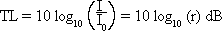We can now construct a table showing the relative intensity levels and transmission losses for cylindrical spreading at various ranges, assuming that r0 is 1 meter.

 Range, r (meters) Relative Intensity, I / I0 Transmission Loss, TL (dB) 1 1 0 10 1/10 10 100 1/100 20 1000 1/1000 30

The relative intensity level decreases less rapidly for cylindrical than for spherical spreading.